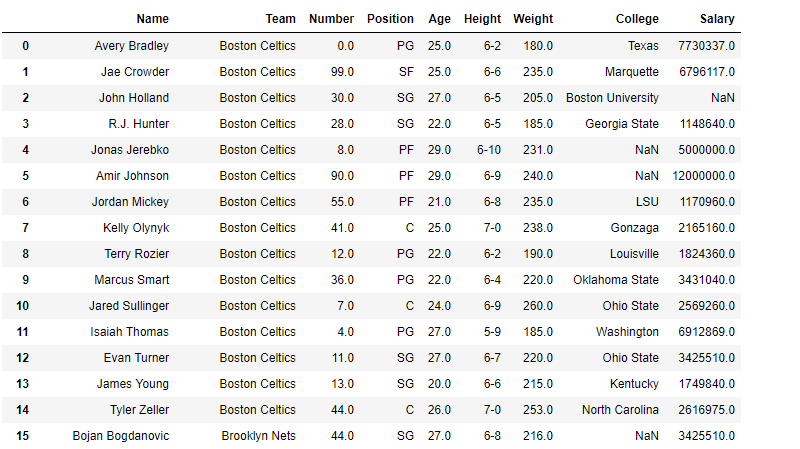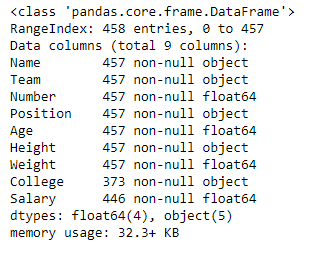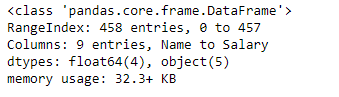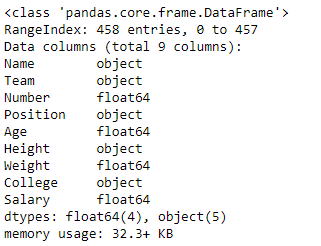# Python | Pandas dataframe.info()

• Last Updated : 25 Aug, 2021

Python is a great language for doing data analysis, primarily because of the fantastic ecosystem of data-centric python packages. Pandas is one of those packages and makes importing and analyzing data much easier.
Pandas dataframe.info() function is used to get a concise summary of the dataframe. It comes really handy when doing exploratory analysis of the data. To get a quick overview of the dataset we use the dataframe.info() function.

Syntax: DataFrame.info(verbose=None, buf=None, max_cols=None, memory_usage=None, null_counts=None)
Parameters :
verbose : Whether to print the full summary. None follows the display.max_info_columns setting. True or False overrides the display.max_info_columns setting.
buf : writable buffer, defaults to sys.stdout
max_cols : Determines whether full summary or short summary is printed. None follows the display.max_info_columns setting.
memory_usage : Specifies whether total memory usage of the DataFrame elements (including index) should be displayed. None follows the display.memory_usage setting. True or False overrides the display.memory_usage setting. A value of ‘deep’ is equivalent of True, with deep introspection. Memory usage is shown in human-readable units (base-2 representation).
null_counts : Whether to show the non-null counts. If None, then only show if the frame is smaller than max_info_rows and max_info_columns. If True, always show counts. If False, never show counts.

Attention geek! Strengthen your foundations with the Python Programming Foundation Course and learn the basics.

To begin with, your interview preparations Enhance your Data Structures concepts with the Python DS Course. And to begin with your Machine Learning Journey, join the Machine Learning - Basic Level Course

Example #1: Use info() function to print full summary of the dataframe.

## Python3

 `# importing pandas as pd``import` `pandas as pd` `# Creating the dataframe``df ``=` `pd.read_csv(``"nba.csv"``)` `# Print the dataframe``df`Let’s print the full summary of the dataframe.

## Python3

 `# to print the full summary``df.info()`

Output :As we can see in the output, the summary includes list of all columns with their data types and the number of non-null values in each column. we also have the value of rangeindex provided for the index axis.

Example #2: Use info() function to print a short summary of the dataframe
Note : In order to print the short summary, we can use the verbose parameter and set it to False.

## Python3

 `# importing pandas as pd``import` `pandas as pd` `# Creating the dataframe``df ``=` `pd.read_csv(``"nba.csv"``)` `# Print the short summary of the``# dataframe by setting verbose = False``df.info(verbose ``=` `False``)`

Output :As, we can see in the output, the summary is very crisp and short. It is helpful when we have 1000s of attributes in dataframe.

Example #3: Use info() function to print a full summary of the dataframe and exclude the null-counts.
Note : In order to print the full summary, with null-counts excluded, we can use null-counts parameter and set it to be false.

## Python3

 `# importing pandas as pd``import` `pandas as pd` `# Creating the dataframe``df ``=` `pd.read_csv(``"nba.csv"``)` `# Print the full summary of the dataframe``# with null count excluded``df.info(verbose ``=` `True``, null_counts ``=` `False``)`

Output :As, we can see in the output, the summary is full but null-counts are excluded.

My Personal Notes arrow_drop_up